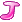# AOJ 0525 Osenbei《挑战程序设计竞赛(第2版)》练习题答案2.1 最基础的“穷竭搜索” 穷竭搜索

AOJ 0525  Osenbei

输入：多组输入，每组第一行为二整数r, c (1 ≤ r ≤ 10, 1 ≤ c ≤ 10 000)，剩下r行c列表示煎饼初始状态。r=c=0表示输入结束。
输出：对于每组输入，输出最多能使多少煎饼正面朝上。

```#include <iostream>
#include <bitset>
#include <algorithm>

using namespace std;

///////////////////////////SubMain//////////////////////////////////
int main(int argc, char *argv[])
{

int R, C;
while(cin >> R >> C && R > 0)
{
int i, j;
for (i = 0; i < R; ++i)
{
for (j = 0; j < C; ++j)
{
bool upwards;
cin >> upwards;
}
}

// 在横向一共有2^R种变换
int permute_r = 1 << R;
int result = 0;
for (i = 0; i < permute_r ; ++i)
{
// 完成当前的变换
for (j = 0; j < R; ++j)
{
// 这一行是否应当翻个面
if (i & (1 << j))
{
}
}

// 对每一列分别算出朝上和朝下的煎饼个数，取其最大值
for (j = 0; j < C; ++j)
{
for (int k = 0; k < R; ++k)
{
{
}
}
}
// 结果取最大值

// 复原
for (j = 0; j < R; ++j)
{
if (i & (1 << j))
{
}
}
}
cout << result << endl;
}

return 0;
}
///////////////////////////End Sub//////////////////////////////////```

### 评论 3

1. #1

博主好厉害，这段代码太妙了
int permute_r = 1 << R;
int result = 0;
for (i = 0; i < permute_r ; ++i)
{
// 完成当前的变换
for (j = 0; j < R; ++j)
{
// 这一行是否应当翻个面
if (i & (1 << j))
{
cookie.flip();
}
}

理解了半天才明白，这样可以在两个循环里面就枚举2^N种情况，不然要我自己想的话，可能要N个循环……每个里面决定是否翻转第N个。

清和6年前 (2017-02-03)回复